Courses

# Soil Mechanics - 8

## 10 Questions MCQ Test GATE Civil Engineering (CE) 2022 Mock Test Series | Soil Mechanics - 8

Description
This mock test of Soil Mechanics - 8 for Civil Engineering (CE) helps you for every Civil Engineering (CE) entrance exam. This contains 10 Multiple Choice Questions for Civil Engineering (CE) Soil Mechanics - 8 (mcq) to study with solutions a complete question bank. The solved questions answers in this Soil Mechanics - 8 quiz give you a good mix of easy questions and tough questions. Civil Engineering (CE) students definitely take this Soil Mechanics - 8 exercise for a better result in the exam. You can find other Soil Mechanics - 8 extra questions, long questions & short questions for Civil Engineering (CE) on EduRev as well by searching above.
QUESTION: 1

### Match the List – I (Boring methods) with List-II (Field conditions) and select the correct answer using the codes given below the lists: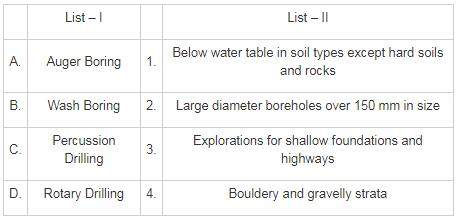Solution:

Percussion boring can be employed in most types of soil, including those containing cobbles and boulders. However, there is generally some disturbance of the soil below the bottom of the borehole, from which samples are taken, and it is extremely difficult to detect thin soil layers and minor geological features with this method.

The advantage of rotary drilling in soils is that progress is much faster than with other investigation methods and disturbance of the soil below the borehole is slight. Also typical core diameters are 41, 54 and 76 mm, but can range up to 165 mm.

Wash boring can be used in most types of soil and below water table but progress becomes slow if particles of coarse gravel size and larger are present.

The test boring using augers can be used for soil exploration work for highways and small structures. Information regarding the types of soil present at various depths is obtained by noting the soil that holds to the auger. The soil samples collected in this manner are disturbed, but they can be used to conduct laboratory tests such as grain size determination and Atterberg limits.

QUESTION: 2

### Consider the following statements if soil is treated with lime. 1. Decrease in shrinkage limit 2. Increase in plastic limit 3. Decrease in liquid limit 4. Flocculation of clay particles Which of the statements given above are correct?

Solution:

When clayey soils with high plasticity are treated with lime, the plasticity index is decreased. The fine clay particles get flocculated and indicate a different grain size distribution. There is slight decrease in liquid limit and considerable increase in plastic limit.

QUESTION: 3

### Which of the followings soil sample may be used to determine consistency limit of the soil?

Solution:

Undisturbed samples → if soil structure, mineral content and moisture content of the soil remains unchanged while sampling then it is called Undisturbed sample.

Representative sample → If soil structure is modified but mineral content and water, content remains unchanged while sampling then it is called representative sample.

Non – Representative Sample → If soil structure mineral content and water content all get modified then it is called non – representative sample.

To determine K, C, ϕ, Cc, Mv undisturbed sample should be used.

To determine consistency limit, specific gravity, particle size analysis either undisturbed or representative sample may be used.

QUESTION: 4

A sampling tube has an inner diameter and outside diameter as shown in the figure below.

D1 = 75 mm

D2 = 80 mm

D3 = 77 mm

D4 = 78 mm

The inside clearance (%) of the samples is

Solution:

Concept:

% inside clearance =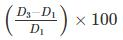where D3 = Inside diameter of sampling tube

D1 = Inside diameter of cutting edge shoe

Calculation: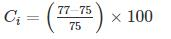Ci = 2.67%

It must lies between 1 to 3%

QUESTION: 5

Consider the following statements:

P. For a clay layer, the net ultimate bearing capacity (By Skempton) at the base of the footing is independent of the cohesion of the soil.

Q. If the depth of the strip footing is equal to the width of the footing, then the value of bearing capacity factor (Nc) by skempton is taken to be 6.0.

R. The theory given by Skempton for net ultimate bearing capacity computations is valid for shallow footings only.

S. Local shear failure occurs in soft clays and loose sands.

Identify the correct option:

Solution:

SKEMPTON’S Method:

This theory is applicable only for C-soils but it can be applied for shallow and deep footings both. In this theory, base resistance and side resistance both are considered. The net ultimate bearing capacity is given by:

qnu=CNc

Where,

Nc = Skempton’s bearing capacity factor which depends upon Df/B ratio

Hence for a clay layer, the net ultimate bearing capacity (By Skempton) at the base of the footing is directly proportional to the cohesion of the soil.

Case 1: When Df/B = 0,

Nc = 5.0 (strip footing)

= 6.0 (Square/Circular/Rectangular/ Raft)

Case 2: When 0 Df/B < 2.5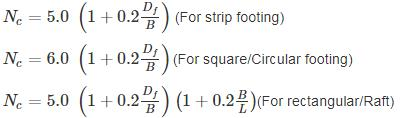Case 3: When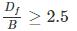Nc = 7.5 (Strip footing)

Nc = 9.0 (Square/circular/rectangular/raft)

Therefore, when B = Df , case 2 is valid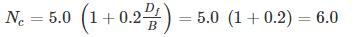Local shear failure:

This type of failure is seen in relatively loose sand and soft clay.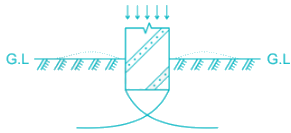Some characteristics of local shear failure are:

1. Failure is not sudden and there is no tilting of footing.

2. Failure surface does not reach the ground surface and slight bulging of soil around the footing is observed

3. Failure surface is not well defined

4. Failure is progressive

5. In load-settlement curve, there is no well-defined peak

6. Failure is characterized by considerable settlement directly beneath the foundation

7. A significant compression of soil below the footing and partial development of plastic equilibrium is observed.

8. Well-defined wedge and slip surfaces only beneath the foundation.

*Answer can only contain numeric values
QUESTION: 6

If the rectangular footing is loaded with eccentric loads having an eccentricity (ex) and (ey) as shown in the figure below, then the effective area taken to calculate the ultimate bearing capacity of soil will be ______ m2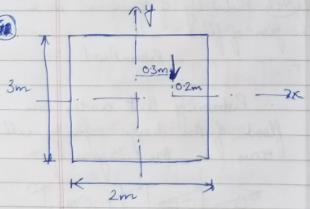Solution:

For eccentric condition, effective area is taken to be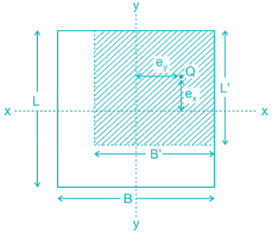Effective area (A') = L' × B'

L' = L – 2ex

B' = B – 2ey

A' = L' × B'

L' = L – 2ex

L' = 3 – 2 × 0.2

L' = 2.6 m

B' = B – 2ey

B' = 2 – 2 × 0.3

B' = 1.4 m

A' = 2.6 × 1.4

A' = 3.64 m2

*Answer can only contain numeric values
QUESTION: 7

A rectangular footing is located at a depth of 1.5 m below the ground surface. It is required to carry the safe load of 1200 kN at the base of the footing. Calculate the area of the footing at the base in m2. Take factor of safety of 2.5 and bearing capacity factors as NC = 38, Nq = 23 and Nγ = 21. Assume water table at a great depth. Use Terzaghi’s ultimate bearing capacity equation. Assume the length to width ratio of the footing to be 1.5. Cohesion c = 100 kN/m2 and unit weight of soil to be 19 kN/m3

Solution:

Concept:

Ultimate bearing capacity given by Terzaghi for rectangular footing is: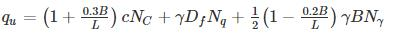Where,

B = width of footing

L = Length of footing

c = Cohesion (kN/m2)

Nc, Nq, Nγ = Bearing capacity factors

γ = unit weight of the soil

Df = Depth of footing

Net ultimate Bearing capacity

qnu = qu - γDf

Net safe Bearing capacity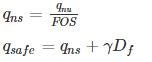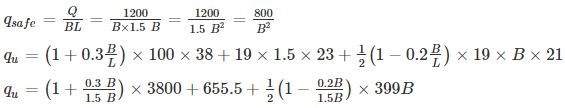qu = 4560 + 655.5 + 172.9 B

qnu = 5215.5 + 172.9 B - γDf

qnu = 5215.5 + 172.9 B – 19 × 1.5

qnu = 5187 + 172.9 B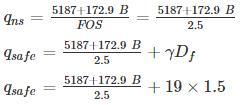qsafe = 2074.8 + 69.16 B + 28.5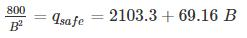800 = (2103.3 + 69.16 B) B2

B = 0.61 m

L = 1.5 × 0.61 = 0.915 m

Area of footing = L x B = 0.558 m2

*Answer can only contain numeric values
QUESTION: 8

A 4m × 3m raft is provided at a depth of 3 m below the ground surface in a purely cohesive soil such that the ultimate bearing capacity by Terzaghi’s is given by 1450kN/m2. The net ultimate bearing capacity by Skempton is _______kN/m2.

Assume Bearing capacity factor by Terzaghi to be: NC = 5.7, Nq = 1.0. Unit weight of clay layer to be 19 kN/m3

Solution:

For raft Foundation [By Terzaghi’s]

Ultimate Bearing Capacity is given by: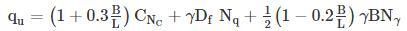For pure clay Nγ = 0

B = 3 m

L = 4 m

NC = 5.7

Nq = 1.0

qu = 1450 kN/m2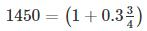C × 5.7 + 19 × 3 × 1.0 + 0

1450 = 1.225 × 5.7 × C + 57

C = 199.49 kN/m2

Now By Skempton:

Net ultimate Bearing capacity is given by:

qnu = CNC

where,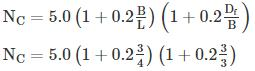Nc = 5.0 × 1.15 × 1.2

NC = 6.9

qnu = CNC

qnu = 199.49 × 6.9

qnu = 1376.48 kN/m2

*Answer can only contain numeric values
QUESTION: 9

A 12 long reinforced concrete pile of diameter 450 mm was driven by drop hammer having total mass of 24000 kg and having an effective fall of 0.9 cm. The average penetration for the last five blows was recorded as 0.6 cm per blow. If the total elastic compression is 2.3 cm, then the safe load on the pile will be _____ kN.

Assume coefficient of restitution and factor of safety to be 0.30 and 2 respectively. Use Hiley’s formula.

Take γconcrete = 25 kN/m3. Efficiency of hammer (ηn) = 1.0.

Solution:

Concept:

By Hiley’s formula

Ultimate load carrying capacity of the pile is given by: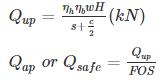Where,

η= efficiency of hammer = 1.0 (drop hammer)
ηb = efficiency of hammer blow =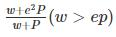e = coefficient of restitution

p = weight of pile

w = weight of hammer

c = constant (elastic compression between pile and soil)

s = set (penetration of pile per blow of hammer)

h = height of fall

calculation: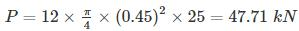W = 24000 × 9.81 = 235.44 kN

ep = 0.30 × 47.71 = 14.31 kN

w > ep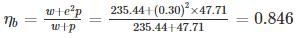ηh = 1.0

s = 0.6 cm/blow

c = 2.3 cm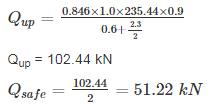*Answer can only contain numeric values
QUESTION: 10

36 Piles are arranged in a square pattern which is embedded in the clayey soil. The shear strength at the base of the pile is 200 kN/m2 and the average shear strength over the depth of the pile is 130 kN/m2. If the Diameter and length of the pile are 0.4 m and 12 m respectively, the safe load that the pile group can carry will be _______ kN. Assume, centre to centre spacing between the pile = 0.8 m, Adhesion factor (α) = 0.50 and Factor of safety = 3

Solution:B = (n – 1)s + d

B = (6 – 1) × 0.8 + 0.4

B = 4.0 + 0.4 = 4.4 m

Ultimate load carrying capacity of single pile:

Qup = qb Ab + qs As

Qb = base resistance of pile = 9c

c = cohesion at base of the pile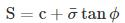ϕ = 0° (clay)

Sbase = c = 200 kN/m2

qs = average skin friction resistance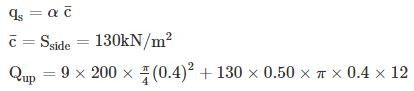Qup = 1206.37 kN

Ultimate load carrying capacity of pile group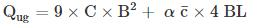= 9 × 200 × (4.4)2 + 0.5 × 130 × 4 × 4.4 × 12

Qug = 48576 kN

Safe load on pile group =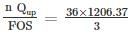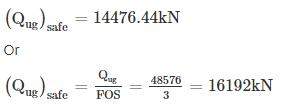So, safe load on pile group will be lesser of two. Hence safe load = 14476.44 kN